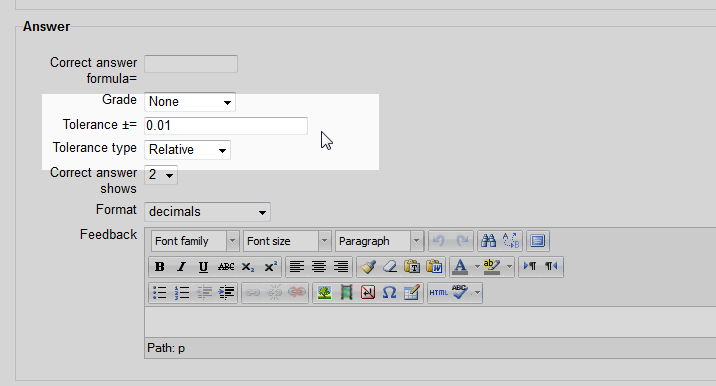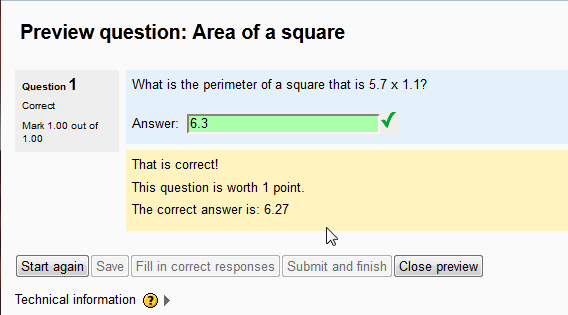# Working with the calculated question Tolerance in Moodle 2.3

In the previous tutorial in on Inserting a Calculated question in the Moodle Question Bank, there was a setting called Tolerance. The Tolerance of a question is used to allow answers to be correct, even if they are close to the correct answer. For example, if a math question is 2.3 x 2.011. The correct answer is 4.6253. If the student answers 4.63, the tolerance can accept that answer as correct, even though its not the exact answer. This is similar to “Grading on a curve“. This tutorial will explain using the tolerance in the Moodle 2.3 calculated question.

### Setting the Tolerance for a calculated question

2. Navigate to the Front page settings > Question bank > Questions. Click one of the calculated questions that were created. If there are no calculated questions create one.
3.On the Editing a Calculated multichoice question page, go to the Answer section. The following chart will explain the different tolerances.

Note! The tolerance is designed to match the answer with a specific parameter. An answer of 15.7 can be answer as 16, 27 as 30, and or 50 as 50.0 and so forth. For example, a Nominal tolerance with the correct answer as 100 and the tolerance as 0.01, the Formula will be (100)*(0.01) <=1. The tolerance is <= 1. So the answer will be correct if its between 99 and 101.

Calculating the tolerance type for correct answers
Tolerance = T
Tolerance±= = X
NominalThe Nominal tolerance will mark an answer correct if the answer is within the parameters of Xc <=T.
RelativeThe Relative tolerance will mark an answer correct if the answer is within the parameters of Xc / c <= T.
Geometric The Geometric tolerance will mark an answer correct if the answer is within the parameters of Xc2 / c2 <= T2

4.Finally, preview the question. The answer should look like the following snapshot. The Tolerance is something that will need to be expirimented with to find the perfect setting for each question.

This concludes the article for Working with the calculated question Tolerance. Please check the next article on Adding a Calculated multichoice question in the Moodle Question Bank. Please visit Working with the Question Bank for more information on questions in the question bank.InMotion Hosting Contributor Content Writer

InMotion Hosting contributors are highly knowledgeable individuals who create relevant content on new trends and troubleshooting techniques to help you achieve your online goals!

More Articles by InMotion Hosting